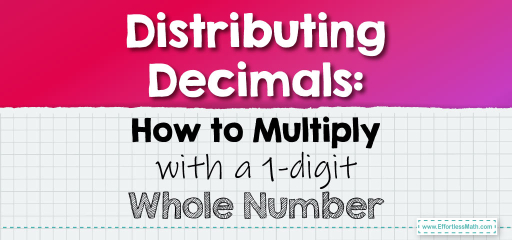# Distributing Decimals: How to Multiply with a 1-digit Whole Number

The distributive property is a fundamental principle in mathematics that states that multiplication distributes over addition. When applied to decimals, it provides a systematic approach to multiplying them by a 1-digit whole number. Let's delve into this method.Distributive Property Formula:

$$a \times (b + c) = (a \times b) + (a \times c)$$

## Multiplying Decimals by a 1-digit Whole Number Using the Distributive Property

### Example 1:

Multiply $$2.3$$ by $$4$$.

Solution Process:

1. Break $$2.3$$ into $$2 + 0.3$$.

2. Apply the distributive property: $$4 \times (2 + 0.3) = (4 \times 2) + (4 \times 0.3)$$.

$$4 \times 2 = 8$$ and $$4 \times 0.3 = 1.2$$. Summing these gives $$9.2$$.

The Absolute Best Book for 5th Grade Students

### Example 2:

Multiply $$3.5$$ by $$3$$.

Solution Process:

1. Break $$3.5$$ into $$3 + 0.5$$.

2. Apply the distributive property: $$3 \times (3 + 0.5) = (3 \times 3) + (3 \times 0.5)$$.

$$3 \times 3 = 9$$ and $$3 \times 0.5 = 1.5$$. Summing these gives $$10.5$$.

The distributive property offers a structured approach to multiplying decimals by 1-digit whole numbers. By breaking down the decimal into its whole number and fractional parts, you can simplify the multiplication process. This method not only reinforces your understanding of decimals but also strengthens your foundational knowledge of multiplication. With practice, you’ll find that using the distributive property makes decimal multiplication a breeze!

### Practice Questions:

1. Multiply $$1.4$$ by $$5$$ using the distributive property.

2. Determine the product of $$2.6$$ and $$6$$ using the distributive property.

3. Multiply $$0.7$$ by $$8$$ using the distributive property.

4. Determine the product of $$3.8$$ and $$7$$ using the distributive property.

5. Multiply $$4.9$$ by $$2$$ using the distributive property.

A Perfect Book for Grade 5 Math Word Problems!

1. $$7$$

2. $$15.6$$

3. $$5.6$$

4. $$26.6$$

5. $$9.8$$

The Best Math Books for Elementary Students

### What people say about "Distributing Decimals: How to Multiply with a 1-digit Whole Number - Effortless Math: We Help Students Learn to LOVE Mathematics"?

No one replied yet.

X
51% OFF

Limited time only!

Save Over 51%

SAVE $15 It was$29.99 now it is \$14.99